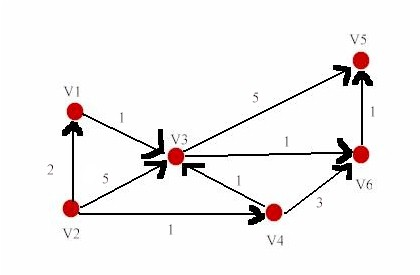Homework #10:

Problem #1:

Apply D-matrix algorithm described in the first handout (available under Cancas | File as ShortestPath_D_Matrix_algorithm.pdf ) to this directed graph.(Please use this fixed vertex order v1, v2, v3, v4, v5, v6 on rows and columns when you put down the matrices.)

(i)               Write down the matrix D(1) ,

(ii)             Calculate and show matrix D(2) from D(1) and D(1),

(iii)          Calculate and show matrix D(3) from D(2) and D(1),

(iv)           Calculate and show matrix D(4) from D(3) and D(1),

(v)             Calculate and show matrix D(5) from D(4) and D(1),

Problem #2:

Apply the F matrix algorithm in the second handout (available under Cancas | File as ShortestPath_F_Matrix_algorithm.pdf ) to the directed graph above to calculate and show the matrices (with the vertex order v1, v2, v3, v4, v5, v6 on rows and columns)

(i)               F(0),

(ii)             F (1),

(iii)          F (2) ,

(iv)           F (3) ,

(v)             F (4) ,

(vi)           F (5) ,

(vii)        F (6).